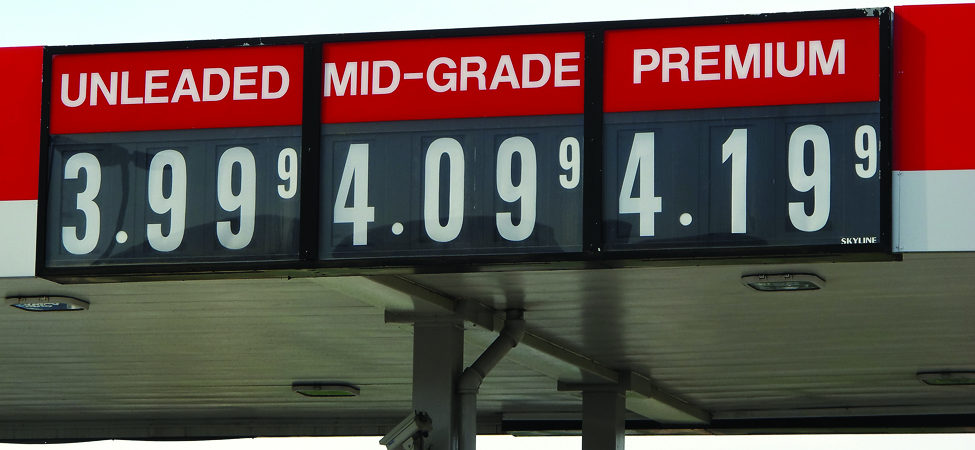## What you’ll learn to do: Write and identify characteristics of decimalsThe price of a gallon of gasoline is written as a decimal number.

Gasoline prices change all the time. They might go down for a period of time, but then they usually rise again. One thing that stays the same is that the price is not usually a whole number. Instead, it is shown using a decimal point to describe the cost in dollars and cents. We use decimal numbers all the time, especially when dealing with money. In this section, we’ll explore decimal numbers and how to perform operations using them.

Before you get started, take this readiness quiz.

1)

Name the number $4,926,015$ in words.

If you missed this problem, review the following example.

Name the number $8,165,432,098,710$ in words.

2)

Round $748$ to the nearest ten.

If you missed this problem, review this video.

3)

If you missed this problem, review the video below.

## Contribute!

Did you have an idea for improving this content? We’d love your input.